Next: About this document ... Up: lab_template Previous: lab_template

Subsections

# Operations on power series

## Purpose

The purpose of this lab is to acquaint you with several useful techniques for working with power series. These techniques include substitution and multiplication and division of series by simple polynomials.

## Getting Started

To assist you, there is a worksheet associated with this lab that contains examples and even solutions to some of the exercises. You can copy that worksheet to your home directory with the following command, which must be run in a terminal window, not in Maple.

cp ~bfarr/Powseries_start.mws ~


You can copy the worksheet now, but you should read through the lab before you load it into Maple. Once you have read to the exercises, start up Maple, load the worksheet Powseries_start.mws, and go through it carefully. Then you can start working on the exercises.

## Background

The general form of a power series inis given below.
The numberis called the base point of the power series. In this lab, we will consider only the special case
. Historically, power series have been used most often to approximate functions that do not have simple formulas. One example is the exponential function, whose power series is given below, along with another power series you have seen in class.

The most familiar example of a power series is the geometric series.
Another example is the power series for(or
), which is
The exercises also use the series forand
, which you haven't seen yet, but will very soon.

Once you have a convergent series representation for a function, it can be manipulated in several ways to generate convergent power series representations of related functions. The rest of the Background describes several different techniques for generating power series representations of functions that are related to power series that are already known. The four techniques are substitution, multiplication and division, integration, and differentiation.

### Substitution

Suppose you have a power series representation for
, and you want a power series representation for
whereis a constant and
is a positive integer The theory for power series says that you can obtain the pwer series you want simply by subsitution. For example, to get a power series for
you just take the series forand substitutefor
, obtainingWriting out the first few terms gives
If you use such a substitution, you have to be careful if the series is only valid for a finite interval. For example, suppose you wanted to find the power series forYou can obtain the desired series by substitution as
but you have to be careful because this formula is not valid for all values of. In fact this formula is only valid if
. The reason for this is that the series for
is only valid ifand when we substitute
for, the formula only makes sense if
.

### Multiplication and Division

If you have a power series representation for, and you want the power series for something like
, you can just multiply each term of the series forby
. If the leading term for the power series representation ofis
for some integer, you can use division to obtain the power series representation for
for any integer
. Some examples follow.
### Term-by-term integration and differentiation

One of the nice properties of power series is that they can be integrated and differentiated term-by-term. For example,
so we have the following power series representation.
Note that the interval of convergence is exactly the same as for the original series, neglecting the behavior at the endpoints.

Integrating a power series term-by-term is very similar, but you may have to include a constant of integration. For example, integrating the power series representation forterm by term gives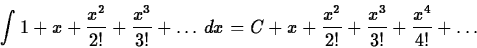You would have to set
to make the right hand side the power series representation for.

## Exercises

1. For the following functions,compare the partial sums of power series up to various orders obtained directly with those obtained by applying addition, subtraction, substitution, multiplication, or division to the power series for,,, or the geometric series for. See the examples in the Getting Started worksheet.
1.
.
2.
.
3.
4.(Hint - start with the series for)
5.2. Use substitution followed by integration to generate the first three terms in the Taylor series with base pointfor
. Start with the series for.

3. In the background section we only considered multiplication of series by polynomials. Suppose you wanted to generate the Taylor polynomial of order ten with base pointfor the function
. Can you do this by multiplying Taylor polynomials forand?Next: About this document ... Up: lab_template Previous: lab_template
William W. Farr
2003-11-14